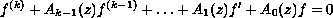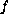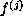Electron. J. Diff. Eqns., Vol. 2004(2004), No. 65, pp. 1-8.

### Finite order solutions of complex linear differential equations Ilpo Laine & Ronghua Yang

Abstract:
We shall consider the growth of solutions of complex linear homogeneous differential equationswith entire coefficients. If one of the intermediate coefficients in exponentially dominating in a sector andis of finite order, then a derivativeis asymptotically constant in a slightly smaller sector. We also find conditions on the coefficients to ensure that all transcendental solutions are of infinite order. This paper extends previous results due to Gundersen and to Belaidi and Hamani.

Submitted December 10, 2003. Published April 28, 2004.
Math Subject Classifications: 30D35, 34M10.
Key Words: Linear differential equations, growth of solutions, iterated order.

Show me the PDF file (215K), TEX file, and other files for this article.Ilpo Laine University of Joensuu, Department of Mathematics P. O. Box 111, FIN-80101 Joensuu, Finland email: ilpo.laine@joensuu.fi Ronghua Yang University of Joensuu, Department of Mathematics P. O. Box 111, FIN-80101 Joensuu, Finland email: ronghua.yang@joensuu.fi